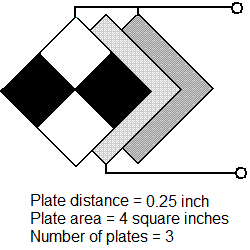# Capacitance Calculator

Area of one plate
square inches

Separation distance
inches

Number of plates (2 or more)

Dielectric constant

Units of Measurement

RESULTS:
Capacitance:

Input Values:
Area:
Separation distance:
Number:
Dielectric Constant:

References:
The ARRL Handbook for Radio CommunicationsTo use the calculator:
1. Choose the units of measurement.
2. Enter the area of one plate.
3. Enter the separation distance.
4. Enter the number of plates.
5. Select the dielectric constant.
6. Press Calculate.

Capacitor with three 4-square-inch platesExample
The diagram shows a capacitor with three 4-square-inch plates, separated by 0.25 inches of air. Its calculated capacitance is 7.18 picofarads.
If instead of air, glass (dielectric constant 7.8) occupies the gap between the plates, the calculated capacitance rises to 55.9 picofarads.

Related Pages:
Capacitive Reactance Calculator
Small Transmitting Loop Antenna